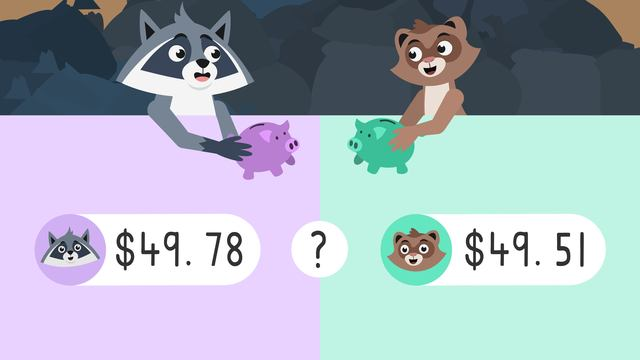# Word Problems: Comparing Money

Content Word Problems: Comparing MoneyRating

Ø 5.0 / 1 ratings
The authorsTeam Digital
Word Problems: Comparing Money
CCSS.MATH.CONTENT.4.MD.A.2

## Comparing Money

Imagine this: You are saving up money for something you want to buy. You check your piggy bank and you want to know how much money you have already saved. To find out, you need to count your money. Let’s figure it out by learning about comparing money.

## What is Comparing Money?

When you compare something, such as money, we are trying to examine the quantities or values to decide if it is greater than, less than, or equal to. We can use the greater than less than or equal to symbols when comparing money.The next section will explain comparing money amounts.

## Comparing Money – Counting Amounts

The first thing you need to do is to count each bill and coin to see how much money you have in your piggy bank. That means we will practice adding and comparing money.

Let’s look at what’s inside Zuri’s piggy bank first and write an equation. Zuri's piggy bank has two twenty dollar bills, a five dollar bill, four dollar bills, three quarters, and three pennies. The illustration below shows the equation:First, add up the dollars, then add up the cents to get the total forty-nine dollars and seventy-eight cents.Now, let’s calculate Freddie’s money. Freddie's piggy bank has a twenty dollar bill, two ten dollar bills, nine dollar bills, three dimes, four nickels, and one penny. The illustration below shows the equation:First, add up the dollars, then add up the cents to get the total forty-nine dollars and fifty-one cents.## Comparing Money – Comparing Values

Now that we know each amount they have, let’s practice comparing money values!

Start by comparing the greatest place value, the tens place. Since they each have a four in the tens place, or forty dollars, we move on to the ones place.Since they each have a nine in the ones place, we move on to the tenths place.In the tenths place Zuri has a seven, and Freddie has a five which means forty-nine dollars and seventy-eight cents is greater than forty-nine dollars and fifty-one cents!## Comparing Money – Summary

Remember, we can use the greater than, less than, or equal to symbols when comparing money. When you want to compare money and you know the amount of money that you have, these steps are helpful:

Step # What to do
2 If the place value digits are equal,
move to the next place value and compare.
3 If the next digits are equal, keep moving
to the next place value until you find
two different numbers to compare.
4 Finally, compare using the greater than,
less than, or equal to symbol.

Want some more practice comparing money after the comparing money video? On this website there is a comparing money amounts worksheet, comparing money worksheets, and comparing money amounts worksheets along with other activities, and interactive exercises.

### TranscriptWord Problems: Comparing Money

1 comment
1 comment
1.This vid was very helpful to me and help me understand the basics of money

From Denique, about 1 year ago

## Word Problems: Comparing Money exercise

Would you like to apply the knowledge you’ve learned? You can review and practice it with the tasks for the video Word Problems: Comparing Money .
• ### Match the symbol to its meaning.

Hints

Remember, the opening faces the larger number, both when we use the greater than symbol and when we use the less than symbol. The opening always faces the bigger number, and we read it left to right.

Look at this example of nine being greater than three: 9 > 3

Solution

These symbols show greater than, less than, and equal to. We see these symbols when we compare numbers.

• ### Add the dollars and cents together.

Hints

Once you have counted the dollars, remember to count up the cents starting at the largest number.

Solution

They earned $7.13. We count 5 + 1 + 1 dollars, so we know the dollar amount is$7.

We count 10 + 1 + 1+ 1 cents, which makes \$00.13 cents.

• ### Use <, >, and = correctly.

Hints

Remember, start by comparing the greatest value. If the largest digit is the same, move to the next place value until you get two different numbers.

Remember, the opening always faces the larger number.

Remember, the = sign means both numbers have equal amounts.

Solution

The greater than sign (>) shows the larger number, the less than sign (<) shows the smaller number, and the equal sign (=) shows the numbers are the same.

• ### How much money does Freddie have?

Hints

Solution

Freddie has __USD__38.51.

His three ten dollar bills equal __USD__30.00, his one five dollar bill equals __USD__5.00, and his three one dollar bills equal __USD__3.00. __USD__30.00 + __USD__5.00 + __USD__3.00 = __USD__38.00

Then, we add the cents. One quarter equals 25 cents, two dimes equals 20 cents, and six pennies equals 6 cents. 25 + 20 + 6 = 51 cents

We add the __USD__38.00 to the 51 cents to make __USD__38.51.

• ### Highlight the question you need to solve.

Hints

Remember, think about what the word problem is asking you to solve.

Look for the question mark (?) to find the question this problem is asking you to solve.

Solution

The question this word problem is asking you to solve is: whose piggy bank has more money? This tells us what to focus on, and that we will be counting and comparing.

Hints